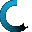Retrieving data ...
Please do not click anywhere else.

If you see no response after 2-3 minutes, then click on the [X] button in the upper right corner to close the window and retry the operation at a later time.
Interactive Multimedia Learning & Teaching Management System Suite## Course Contents

Course Name: Elementary Subtraction - Online
• Elementary Subtraction
•  1.1 Introduction to Subtraction
•  1.2 Subtraction Using Objects
•  1.3 Identity Property of Subtraction
•  1.4 Subtraction in Relation to Addition
•  1.5 Subtraction Using a Subtraction Table
•  1.6 Subtraction Using Regrouping
•  1.7 Find 10 Less
•  1.8 True or False
•  1.9 Find the Missing Number
•  1.10 Subtraction with Borrowing Numbers
•  1.11 Subtracting a 1-digit Number from a 2-digit Number
•  1.12 Subtracting a 2-digit Number from a 2-digit Number
•  1.13 Subtracting a 1-digit Number from a 3-digit Number
•  1.14 Subtracting a 2-digit Number from a 3-digit Number
•  1.15 Subtracting a 3-digit Number from a 3-digit Number